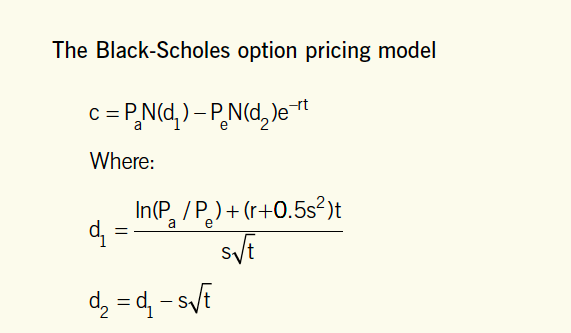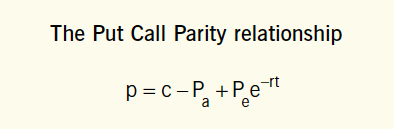AFMP4
Syllabus B. Advanced Investment Appraisal B2. Application of option pricing theory in investment decisions

# B2a. Black-Scholes Option Pricing (BSOP) model 2 / 7

### Syllabus B2a)

Apply the Black-Scholes Option Pricing (BSOP) model to financial product valuation and to asset valuation:

i) Determine and discuss, using published data, the five principal drivers of option value (value of the underlying, exercise price, time to expiry, volatility and the risk- free rate)

ii) Discuss the underlying assumptions, structure, application and limitations of the BSOP model.

### Black-Scholes model is a model for determining the price of a call option

The market value of a call option can be calculated as:#### Note:

The formula will be given in the examination paper.

You need to be aware only of the variables which it includes, to be able to plug in the numbers.

The key:

• Pa = current price of underlying asset (e.g. share price)

Pe = exercise price

r = risk­ free rate of interest

t = time until expiry of option in years

s = volatility of the share price (as measured by the standard deviation expressed as a decimal)

N(d) = equals the area under the normal curve up to d (see normal distribution tables)

e = 2.71828, the exponential constant

In = the natural log (log to be base e)

#### Illustration

The current share price of AA plc is £2.90.
Estimate the value of a call option on the share of the company, with an exercise price of £2.60, and 6 months to run before it expires.

The risk free rate of interest is 6% and the variance of the rate of return on the shares has been 15%.

#### Solution

• d1 = (ln(2.9 / 2.6) + (0.06 × 0.5 × 0.15)) / (√0.15 × √0.5)
d1 = 0.6452, approximate to two decimal places = 0.65
d1 = 0.65 – (√0.15 x√0.5)
d1 = 0.3713 rounded to 0.37

• Using the normal distribution table:

Nd1 = N( 0.65) = 0.5 + 0.24 = 0.74
Nd2 = N(0.37) = 0.5 + 0.14 = 0.64

• Using calculator

e^(-rt) = e^(-0.03)
e^(-rt) = 0.97

• Call option price = (2.90 x 0.74) – (2.60 x 0.97 x 0.64) = £0.53

#### Using the Black-Scholes model to value put options

The put call parity equation is on the examination formula sheet:#### Steps:

1. Step 1: Value the corresponding call option using the Black-Scholes model.

2. Step 2: Then calculate the value the put option using the put call parity equation.

#### Illustration (continue)

• P = 0.53 – 2.9 + 2.60 (0.97)
P = £0.15

#### Underlying assumptions and limitations

The model assumes that:

• The options are European calls.

• There are no transaction costs or taxes.

• The investor can borrow at the risk free rate.

• The risk free rate of interest and the share’s volatility is constant over the life of the option.

• The future share price volatility can be estimated by observing past share price volatility.

• The share price follows a random walk and that the possible share prices are based on a normal distribution.

• No dividends are payable before the option expiry date.

#### Application to American call options

• One of the limitations of the Black-Scholes formula is that it assumes that the shares will not pay dividends before the option expires.

If this holds true then the model can also be used to value American call options.

• In fact, if no dividends are payable before the option expiry date, the American call option will be worth the same as a European call option.

• You will not be asked to value American call options on shares that do pay dividends or American put options using the Black­Scholes model.

#### Application to shares where dividends are payable before the expiry date

The Black­Scholes formula can be adapted to call options with dividends being paid before expiry by calculating a ‘dividend adjusted share price’:

• Simply deduct the present value of dividends to be paid (before the expiry of the option) from the current share price.

• Pa, becomes Pa – PV (dividends) in the Black-Scholes formula.

PV of dividend = De-rt

Where D = dividend

#### Illustration

The following information relates to a call option:
Current share price £60
Exercise price £70
Dividend to be paid in 3 month time £1.5
Risk free rate 5%
Expiry date is 5 months.

• The dividend-adjusted share price for Black-Scholes option pricing model can be calculated as:

PV of dividend = De-rt

r = 0.05
t = 3/12 = 0.25 of a year

PV of dividend =1.5 e-(0.05 x 0.25)
PV of dividend = £1.48

Dividend-adjusted price = 60 –1.48 = £58.52 and this will replace the price of the underlying item in the formula.